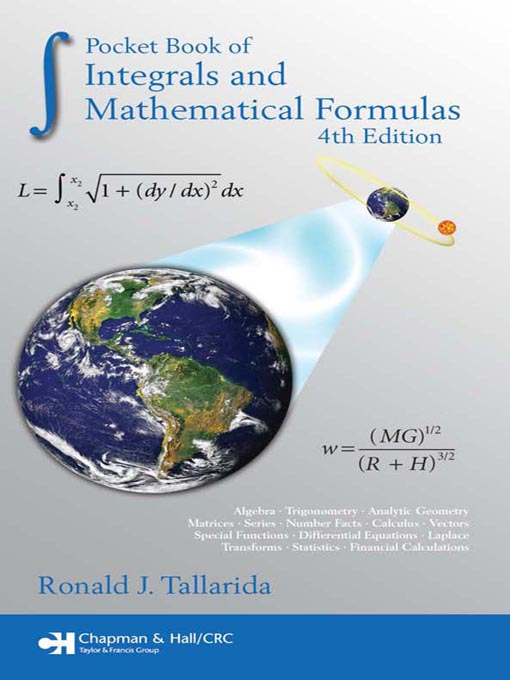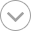Try refreshing the page. If that doesn't work, there may be a network issue, and you can use our self test page to see what's preventing the page from loading.
No sample available# Pocket Book of Integrals and Mathematical Formulas

ebook

PDF ebook

## Languages

English

Convenient Organization of Essential Material so You Can Look up Formulas Fast

Containing a careful selection of standard and timely topics, the Pocket Book of Integrals and Mathematical Formulas, Fourth Edition presents many numerical and statistical tables, scores of worked examples, and the most useful mathematical formulas for engineering and scientific applications. This fourth edition of a bestseller provides even more comprehensive coverage with the inclusion of several additional topics, all while maintaining its accessible, clear style and handy size.

New to the Fourth Edition

• An expanded chapter on series that covers many fascinating properties of the natural numbers that follow from number theory
• New applications such as geostationary satellite orbits and drug kinetics
• An expanded statistics section that discusses nonlinear regression as well as the normal approximation of the binomial distribution
• Revised format of the table of integrals for easier use of the forms and functions

Easy to Use on the Go

The book addresses a range of areas, from elementary algebra, geometry, matrices, and trigonometry to calculus, vector analysis, differential equations, and statistics. Featuring a convenient, portable size, it is sure to remain in the pockets or on the desks of all who use mathematical formulas and tables of integrals and derivatives.

•